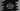# C++ program to print right-angled right-oriented pyramid of numbers## C++ program to print right-angled right-oriented pyramid of numbers:

In this post, we will learn how to print a right-oriented right angled triangle of numbers. I will show you how to remember the algorithm to print the pattern and how to write the program from the algorithm.

The program will take the height of the Pyramid as an input from the user and it will print that pyramid of that height.

### What is a right-angled right-oriented pyramid:

A right-angled number pyramid looks as like below:

``````1
2    3
4    5    6
7    8    9    10
11  12   13    14   15``````

A right-oriented right-angled triangle is just its opposite.

``````                        1
2    3
4    5    6
7    8    9   10
11   12   13   14   15``````

Let’s learn how to write the algorithm before we start to code:

### Algorithm to print a right-angled right-oriented pyramid:

If you look closely, you will see that we are printing blank spaces or a number. Let me add a * where a blank space is printed:

``````    *    *    *    *    1
*    *    *    2    3
*    *    4    5    6
*    7    8    9   10
11   12   13   14   15``````

Here,

• The height of the pyramid is 5
• For the line 1, we are printing 4 stars.
• For the line 2, we are printing 3 stars, etc. Once the stars are printed, we are printing the numbers.

We can use the following algorithm to write this program:

• Take the height as an input from the user.
• Initialize a variable as 1 to hold the number to print.
• Run a for loop to print each row of the pyramid.

• Inside the loop, use another for loop to print the blank spaces.
• Use another for loop to print the numbers. Increment the value of the number variable by 1 after each print.
• Move to a new line after each line.
• Also, we have to make sure that we are printing equal number of characters including the blank spaces and the number. Otherwise, the numbers will not be aligned vertically.

Let’s write down the program:

### C++ program to print a right-angled right-oriented pyramid:

Below is the complete program:

``````#include <iostream>
#include <iomanip>
using namespace std;

int main()
{

int number = 1, i, j, k, rows;

cout << "Enter the rows: ";
cin >> rows;

for (i = 1; i <= rows; i++)
{
for (j = i; j < rows; j++)
{
cout << setw(5) << "";
}
for (k = 1; k <= i; k++)
{
cout << setw(5) << number;
number++;
}
cout << endl;
}

return 0;
}``````

Here,

• number is an integer variable to hold the number value to print in the triangle. rows is another integer variable to hold the total rows count.
• It asks the user to enter the row value and reads that value to the variable rows.
• The for loop with i runs to print each row of the triangle. It runs from i = 1 to rows.

• The first inner for loop prints the starting blank spaces for each row. We are using setw(5) to set the width of the blank spaces to 5.
• The second inner for loop prints the value of the number. The setw(5) statement ensures that the total width of the print is always 5. So, if it prints a single digit number, it adds four blank spaces to its left and if it prints a double digit number, it adds three blank spaces to its left. It will keep all numbers vertically aligned.
• After the number is printed, we are incrementing its value by 1.
• At the end of the loop, add a new line.

If you run this program, it will print a right-oriented right-angled triangle for a given height.

### Sample output:

If you run the program, it will print output as like below:

``````Enter the rows: 10
1
2    3
4    5    6
7    8    9   10
11   12   13   14   15
16   17   18   19   20   21
22   23   24   25   26   27   28
29   30   31   32   33   34   35   36
37   38   39   40   41   42   43   44   45
46   47   48   49   50   51   52   53   54   55

Enter the rows: 5
1
2    3
4    5    6
7    8    9   10
11   12   13   14   15

Enter the rows: 15
1
2    3
4    5    6
7    8    9   10
11   12   13   14   15
16   17   18   19   20   21
22   23   24   25   26   27   28
29   30   31   32   33   34   35   36
37   38   39   40   41   42   43   44   45
46   47   48   49   50   51   52   53   54   55
56   57   58   59   60   61   62   63   64   65   66
67   68   69   70   71   72   73   74   75   76   77   78
79   80   81   82   83   84   85   86   87   88   89   90   91
92   93   94   95   96   97   98   99  100  101  102  103  104  105
106  107  108  109  110  111  112  113  114  115  116  117  118  119  120``````